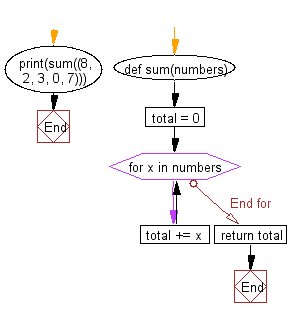﻿ Python Exercise: Find the sum of all the numbers in a list - w3resource# Python Exercise: Find the sum of all the numbers in a list

## Python Functions: Exercise-2 with Solution

Write a Python function to sum all the numbers in a list.

Sample Solution:-

Python Code:

``````def sum(numbers):
total = 0
for x in numbers:
total += x
print(sum((8, 2, 3, 0, 7)))
```
```

Sample Output:

```20
```

Pictorial presentation:Flowchart:## Visualize Python code execution:

The following tool visualize what the computer is doing step-by-step as it executes the said program:

Python Code Editor:

Have another way to solve this solution? Contribute your code (and comments) through Disqus.

What is the difficulty level of this exercise?

Test your Programming skills with w3resource's quiz.

﻿

## Python: Tips of the Day

Decapitalizes the first letter of a string:

Example:

```def tips_decapitalize(s, upper_rest=False):
return s[:1].lower() + (s[1:].upper() if upper_rest else s[1:])
print(tips_decapitalize('PythonTips'))
print(tips_decapitalize('PythonTips', True))
```

Output:

```pythonTips
pYTHONTIPS
```